# Python | Linear Regression using sklearn

Prerequisite: Linear Regression

Linear Regression is a machine learning algorithm based on supervised learning. It performs a regression task. Regression models a target prediction value based on independent variables. It is mostly used for finding out the relationship between variables and forecasting. Different regression models differ based on – the kind of relationship between dependent and independent variables, they are considering and the number of independent variables being used.

This article is going to demonstrate how to use the various Python libraries to implement linear regression on a given dataset. We will demonstrate a binary linear model as this will be easier to visualize.

In this demonstration, the model will use Gradient Descent to learn. You can learn about it here.

Step 1: Importing all the required libraries

 `import` `numpy as np ` `import` `pandas as pd ` `import` `seaborn as sns ` `import` `matplotlib.pyplot as plt ` `from` `sklearn ``import` `preprocessing, svm ` `from` `sklearn.model_selection ``import` `train_test_split ` `from` `sklearn.linear_model ``import` `LinearRegression `

 `cd C:\Users\Dev\Desktop\Kaggle\Salinity ` ` `  `# Changing the file read location to the location of the dataset ` `df ``=` `pd.read_csv(``'bottle.csv'``) ` `df_binary ``=` `df[[``'Salnty'``, ``'T_degC'``]] ` ` `  `# Taking only the selected two attributes from the dataset ` `df_binary.columns ``=` `[``'Sal'``, ``'Temp'``] ` ` `  `# Renaming the columns for easier writing of the code ` `df_binary.head() ` ` `  `# Displaying only the 1st  rows along with the column names `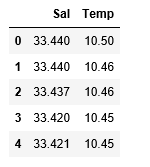Step 3: Exploring the data scatter

 `sns.lmplot(x ``=``"Sal"``, y ``=``"Temp"``, data ``=` `df_binary, order ``=` `2``, ci ``=` `None``) ` ` `  `# Plotting the data scatter `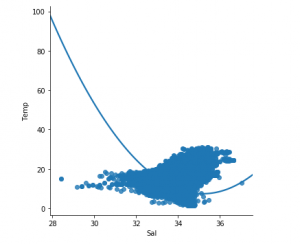Step 4: Data cleaning

 `# Eliminating NaN or missing input numbers ` `df_binary.fillna(method ``=``'ffill'``, inplace ``=` `True``) `

Step 5: Training our model

 `X ``=` `np.array(df_binary[``'Sal'``]).reshape(``-``1``, ``1``) ` `y ``=` `np.array(df_binary[``'Temp'``]).reshape(``-``1``, ``1``) ` ` `  `# Separating the data into independent and dependent variables ` `# Converting each dataframe into a numpy array  ` `# since each dataframe contains only one column ` `df_binary.dropna(inplace ``=` `True``) ` ` `  `# Dropping any rows with Nan values ` `X_train, X_test, y_train, y_test ``=` `train_test_split(X, y, test_size ``=` `0.25``) ` ` `  `# Splitting the data into training and testing data ` `regr ``=` `LinearRegression() ` ` `  `regr.fit(X_train, y_train) ` `print``(regr.score(X_test, y_test)) `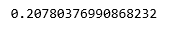Step 6: Exploring our results

 `y_pred ``=` `regr.predict(X_test) ` `plt.scatter(X_test, y_test, color ``=``'b'``) ` `plt.plot(X_test, y_pred, color ``=``'k'``) ` ` `  `plt.show() ` `# Data scatter of predicted values `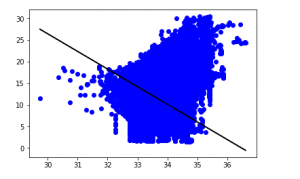The low accuracy score of our model suggests that our regressive model has not fitted very well to the existing data. This suggests that our data is not suitable for linear regression. But sometimes, a dataset may accept a linear regressor if we consider only a part of it. Let us check for that possibility.

Step 7: Working with a smaller dataset

 `df_binary500 ``=` `df_binary[:][:``500``] ` ` `  `# Selecting the 1st 500 rows of the data ` `sns.lmplot(x ``=``"Sal"``, y ``=``"Temp"``, data ``=` `df_binary500, ` `                               ``order ``=` `2``, ci ``=` `None``) `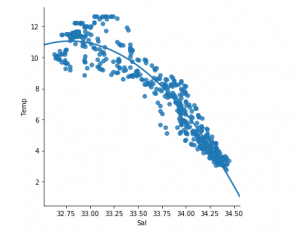We can already see that the first 500 rows follow a linear model. Continuing with the same steps as before.

 `df_binary500.fillna(method ``=``'ffill'``, inplace ``=` `True``) ` ` `  `X ``=` `np.array(df_binary500[``'Sal'``]).reshape(``-``1``, ``1``) ` `y ``=` `np.array(df_binary500[``'Temp'``]).reshape(``-``1``, ``1``) ` ` `  `df_binary500.dropna(inplace ``=` `True``) ` `X_train, X_test, y_train, y_test ``=` `train_test_split(X, y, test_size ``=` `0.25``) ` ` `  `regr ``=` `LinearRegression() ` `regr.fit(X_train, y_train) ` `print``(regr.score(X_test, y_test)) `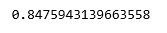`y_pred ``=` `regr.predict(X_test) ` `plt.scatter(X_test, y_test, color ``=``'b'``) ` `plt.plot(X_test, y_pred, color ``=``'k'``) ` ` `  `plt.show() `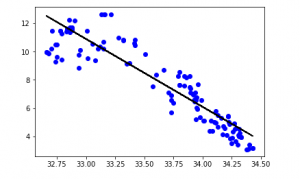My Personal Notes arrow_drop_upCheck out this Author's contributed articles.

If you like GeeksforGeeks and would like to contribute, you can also write an article using contribute.geeksforgeeks.org or mail your article to contribute@geeksforgeeks.org. See your article appearing on the GeeksforGeeks main page and help other Geeks.

Please Improve this article if you find anything incorrect by clicking on the "Improve Article" button below.

Improved By : shubham_singh

Article Tags :
Practice Tags :

1

Please write to us at contribute@geeksforgeeks.org to report any issue with the above content.